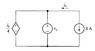# Electric Circuits: Find Vg & Why is it -2V?

• sevag00

## Homework Statement

Hi. I have an electric circuit attached below. The question is to find the value Vg in order for the interconnection to be valid. The answer is -2V.
My question is, why is the voltage minus?

#### Attachments

•Capture.JPG
4.8 KB · Views: 352
You did the calculation - if you read the diagram correctly you set the [+] terminal to have a higher voltage than the [-] terminal. The minus sign means that the terminals are drawn the wrong way around.
As to "why", you may have done the calculation wrong or maybe that's just the way the physics works out for that configuration.

You did the calculation - if you read the diagram correctly you set the [+] terminal to have a higher voltage than the [-] terminal. The minus sign means that the terminals are drawn the wrong way around.
As to "why", you may have done the calculation wrong or maybe that's just the way the physics works out for that configuration.

## Homework Statement

Hi. I have an electric circuit attached below. The question is to find the value Vg in order for the interconnection to be valid. The answer is -2V.
My question is, why is the voltage minus?

Show how you would determine the potential of the controlled source on the left.

ib=8A, Vx= ib/4 = 8/4=2V

Last edited:
ib=8A, Vx= ib/4 = 8/4=2V

Check the defined direction of ib. Is the 8 A source really driving current in the direction indicated?

Oh. You mean they are in opposite direction. So the current ib is negative, right?

Oh. You mean they are in opposite direction. So the current ib is negative, right?

Right.

Good. Thanks for the help.